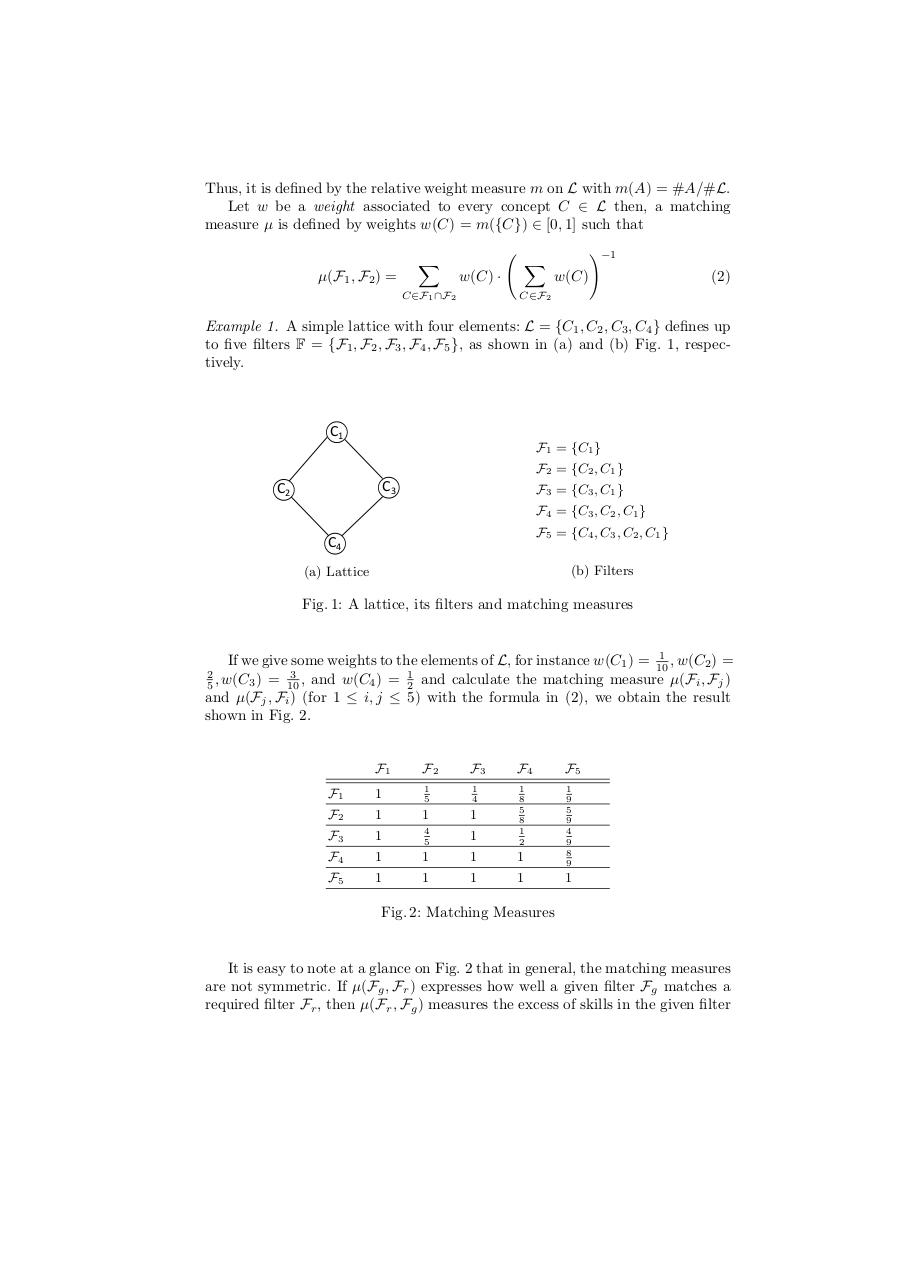# Top K Queries.pdfPage 1 2 3 45615

#### Text preview

Thus, it is defined by the relative weight measure m on L with m(A) = #A/#L.
Let w be a weight associated to every concept C ∈ L then, a matching
measure µ is defined by weights w(C) = m({C}) ∈ [0, 1] such that
µ(F1 , F2 ) =

X

X

w(C) ·

C∈F1 ∩F2

!−1

w(C)

C∈F2

(2)

Example 1. A simple lattice with four elements: L = {C1 , C2 , C3 , C4 } defines up
to five filters F = {F1 , F2 , F3 , F4 , F5 }, as shown in (a) and (b) Fig. 1, respectively.

C1
F1 = {C1 }
F2 = {C2 , C1 }

C3

C2

F3 = {C3 , C1 }
F4 = {C3 , C2 , C1 }
F5 = {C4 , C3 , C2 , C1 }

C4

(b) Filters

(a) Lattice

Fig. 1: A lattice, its filters and matching measures

1
If we give some weights to the elements of L, for instance w(C1 ) = 10
, w(C2 ) =
1
= 2 and calculate the matching measure µ(Fi , Fj )
≤ 5) with the formula in (2), we obtain the result
shown in Fig. 2.
2
3
5 , w(C3 ) = 10 , and w(C4 )
and µ(Fj , Fi ) (for 1 ≤ i, j

F1

F2

F3

F4

F5

F1

1

1
5

1
4

F2

1

1

1

F3

1

4
5

1

1
8
5
8
1
2

F4

1

1

1

1

1
9
5
9
4
9
8
9

F5

1

1

1

1

1

Fig. 2: Matching Measures

It is easy to note at a glance on Fig. 2 that in general, the matching measures
are not symmetric. If µ(Fg , Fr ) expresses how well a given filter Fg matches a
required filter Fr , then µ(Fr , Fg ) measures the excess of skills in the given filter